$$Accuracy = \frac{n_ }{n_}$$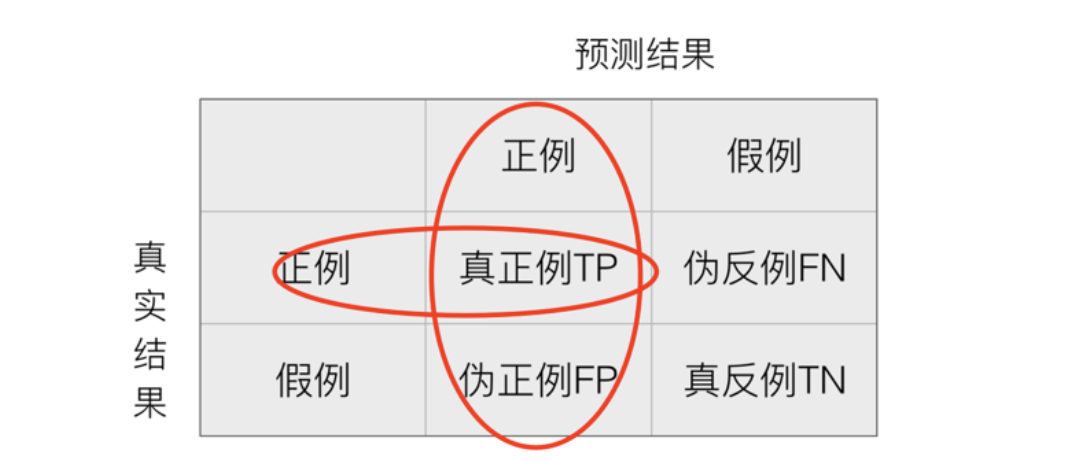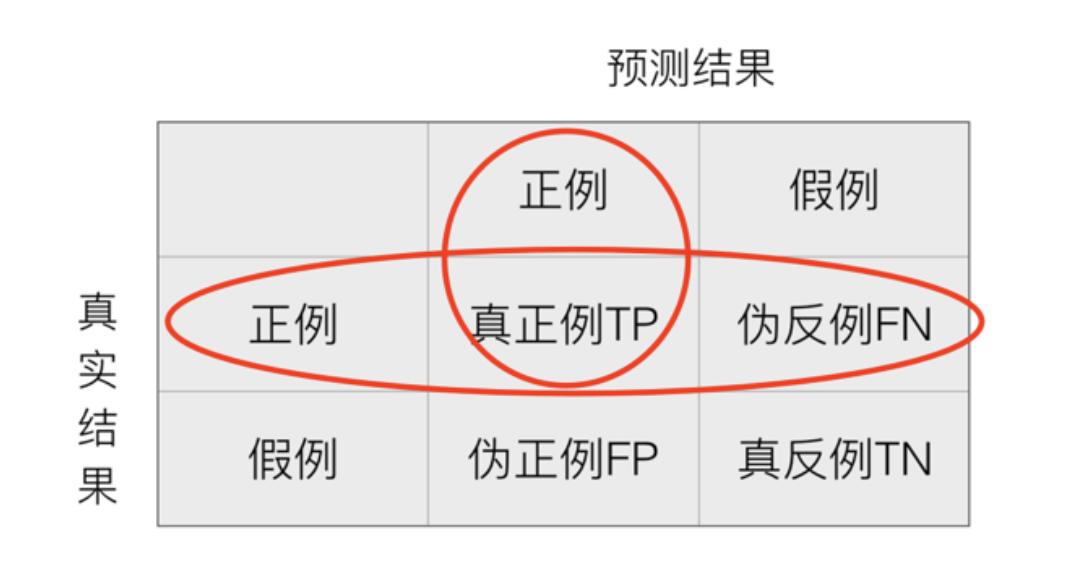F1 score

F1 score是精确率和召回率的调和平均值

$$\begin &= \frac{2TP}{2TP + FN + FP} \endF1 &= \frac{2*precision * recall}{precision + recall} \$$

P-R曲线

P-R曲线的横轴是召回率，纵轴是精确率。对于一个排序模型来说，其P-R曲线上的一个点代表着，在某一阈值下，模型将大于该阈值的结果判定为正样本， 小于该阈值的结果判定为负样本，此时返回结果对应的召回率和精确率。整条P-R 曲线是通过将阈值从高到低移动而生成的。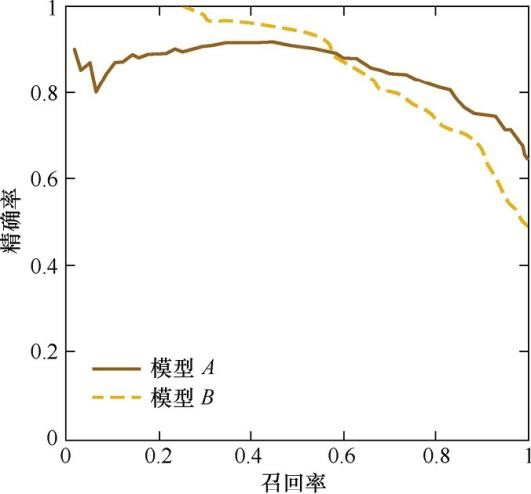RMSE

$$RMSE = \sqrt{\sum_ ^n (y_i - \hat y_i)}$$

ROC曲线

ROC曲线是Receiver Operating Characteristic Curve的简称，中文名为“受试者工作特征曲线”。ROC曲线源于军事领域，而后在医学领域应用甚广，“受试者工作 特征曲线”这一名称也正是来自于医学领域。

ROC曲线的横坐标为假阳性率（False Positive Rate，FPR）；纵坐标为真阳性 率（True Positive Rate，TPR）。FPR和TPR的计算方法分别为

\begin{align*} FPR = \frac = \frac TPR = \frac{TP + FN} \ = \frac{TN+FP} \end{align*}

ROC曲线是通过不断移动分类器的“截断点”来生成曲线上的一组关 键点的，通过动态地调整截断点，从最高的得分开始（实际上是从正无穷开始，对应 着ROC曲线的零点），逐渐调整到最低得分，每一个截断点都会对应一个FPR和 TPR，在ROC图上绘制出每个截断点对应的位置，再连接所有点就得到最终的 ROC曲线。

AUC

AUC指的是ROC曲线下的面积大小，该值能够量化地反映基于 ROC曲线衡量出的模型性能。计算AUC值只需要沿着ROC横轴做积分就可以了。 由于ROC曲线一般都处于$y=x$这条直线的上方（如果不是的话，只要把模型预测的 概率反转成1−p就可以得到一个更好的分类器），所以AUC的取值一般在0.5～1之 间。AUC越大，说明分类器越可能把真正的正样本排在前面，分类性能越好。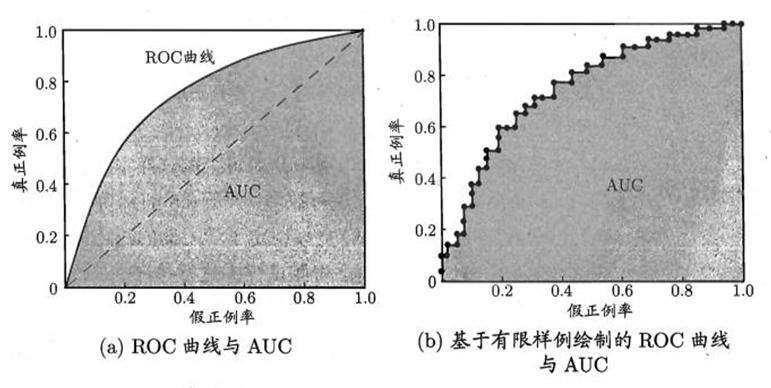• 加载数据集
## Load Data and train model
# 对于本示例，我们从 OpenML <https://www.openml.org/d/1464> 加载输血服务中心数据集。这是一个二元分类问题，目标是个人是否献血。然后将数据拆分为训练数据集和测试数据集，并使用训练数据集拟合逻辑回归。

from sklearn.datasets import fetch_openml
from sklearn.preprocessing import StandardScaler
from sklearn.pipeline import make_pipeline
from sklearn.linear_model import LogisticRegression
from sklearn.model_selection import train_test_split

X, y = fetch_openml(data_id=1464, return_X_y=True)
X_train, X_test, y_train, y_test = train_test_split(X, y, stratify=y)

clf = make_pipeline(StandardScaler(), LogisticRegression(random_state=0))
clf.fit(X_train, y_train)

• 展示混淆矩阵
# 使用拟合模型，我们计算模型对测试数据集的预测。这些预测用于计算使用 :class:ConfusionMatrixDisplay 绘制的混淆矩阵
from sklearn.metrics import confusion_matrix
from sklearn.metrics import ConfusionMatrixDisplay

y_pred = clf.predict(X_test)
cm = confusion_matrix(y_test, y_pred)

cm_display = ConfusionMatrixDisplay(cm).plot()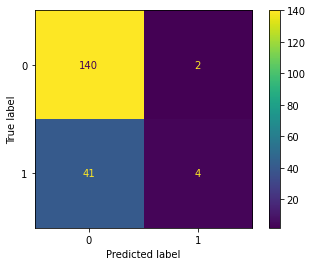• 绘制ROC曲线
# roc 曲线需要来自估计器的概率或非阈值决策值。由于逻辑回归提供了一个决策函数，我们将使用它来绘制 roc 曲线：
from sklearn.metrics import roc_curve
from sklearn.metrics import RocCurveDisplay
y_score = clf.decision_function(X_test)

fpr, tpr, _ = roc_curve(y_test, y_score, pos_label=clf.classes_)
roc_display = RocCurveDisplay(fpr=fpr, tpr=tpr).plot()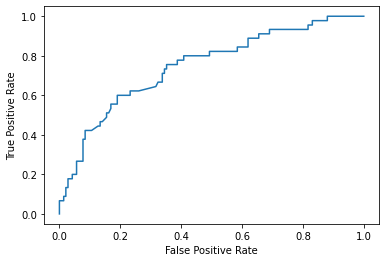• 绘制P-R曲线
from sklearn.metrics import precision_recall_curve
from sklearn.metrics import PrecisionRecallDisplay

prec, recall, _ = precision_recall_curve(y_test, y_score,
pos_label=clf.classes_)
pr_display = PrecisionRecallDisplay(precision=prec, recall=recall).plot()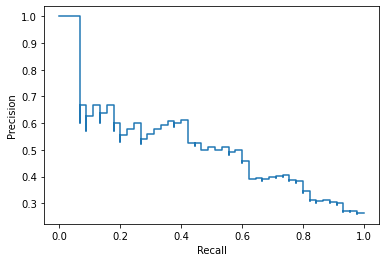• 联合展示
import matplotlib.pyplot as plt
fig, (ax1, ax2) = plt.subplots(1, 2, figsize=(12, 8))

roc_display.plot(ax=ax1)
pr_display.plot(ax=ax2)
plt.show()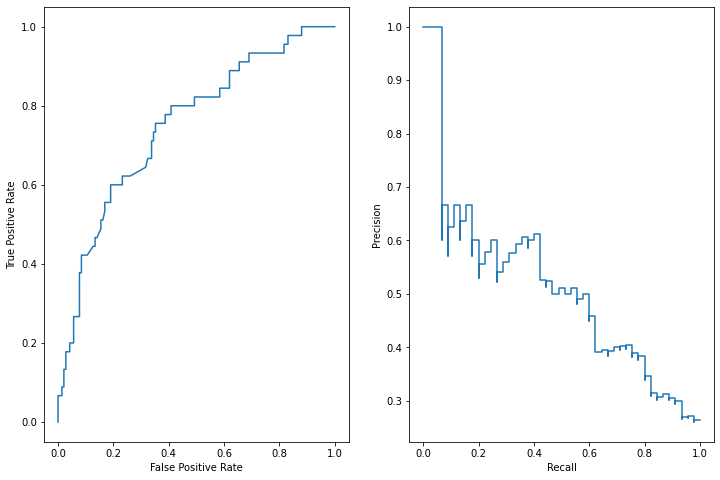• sklearn中提供了非常多的算法实现和示例代码，用户只要查阅相关API即可，可节约时间
• 其实还有很多的模型评估方法，例如西瓜书中还提到了代价敏感错误率与代价曲线，有兴趣的读者可进一步了解